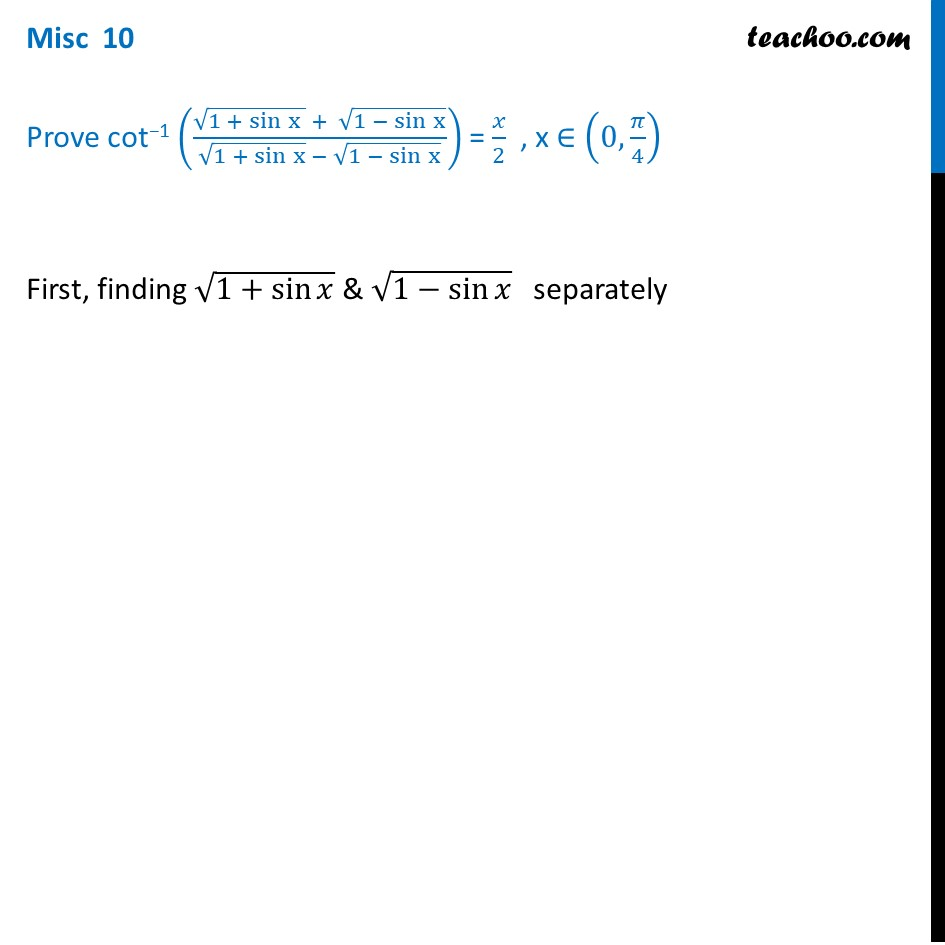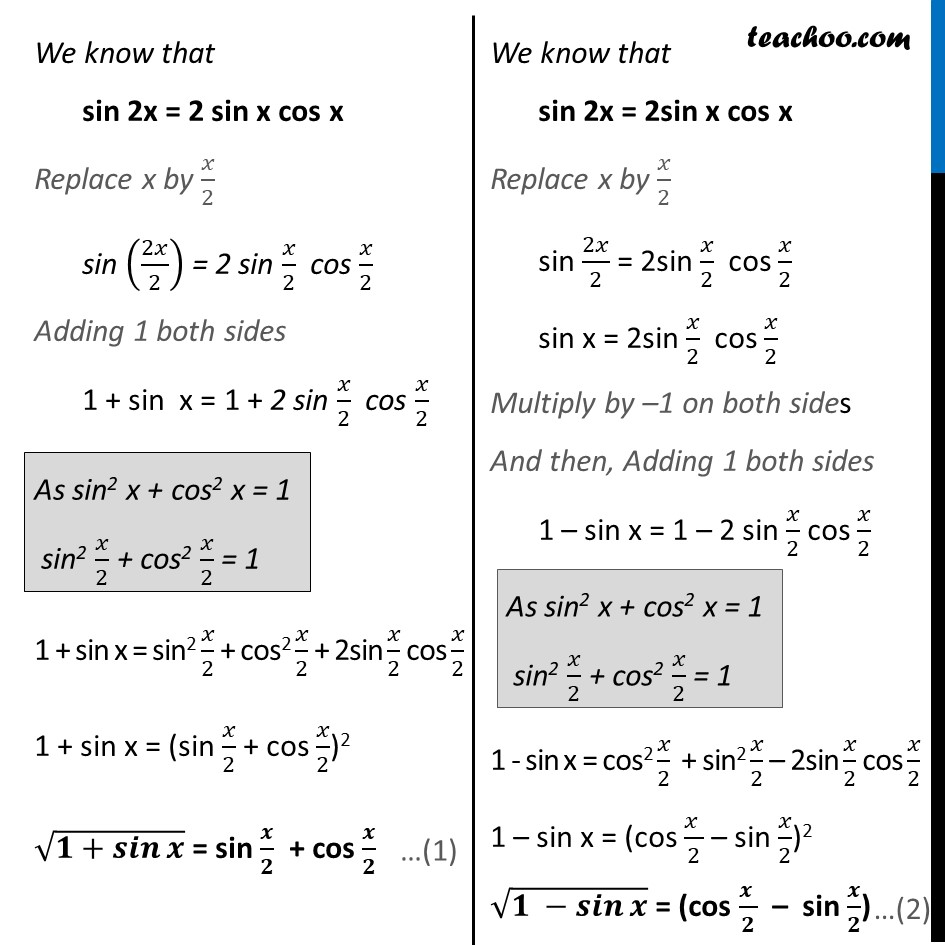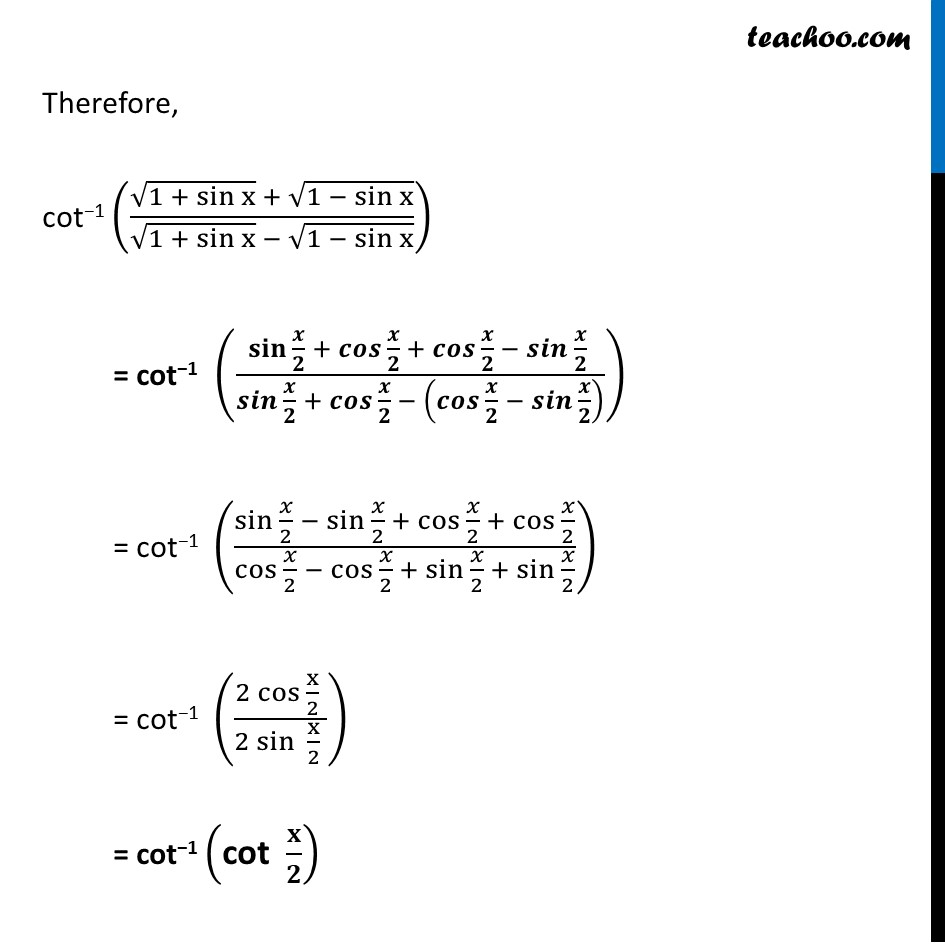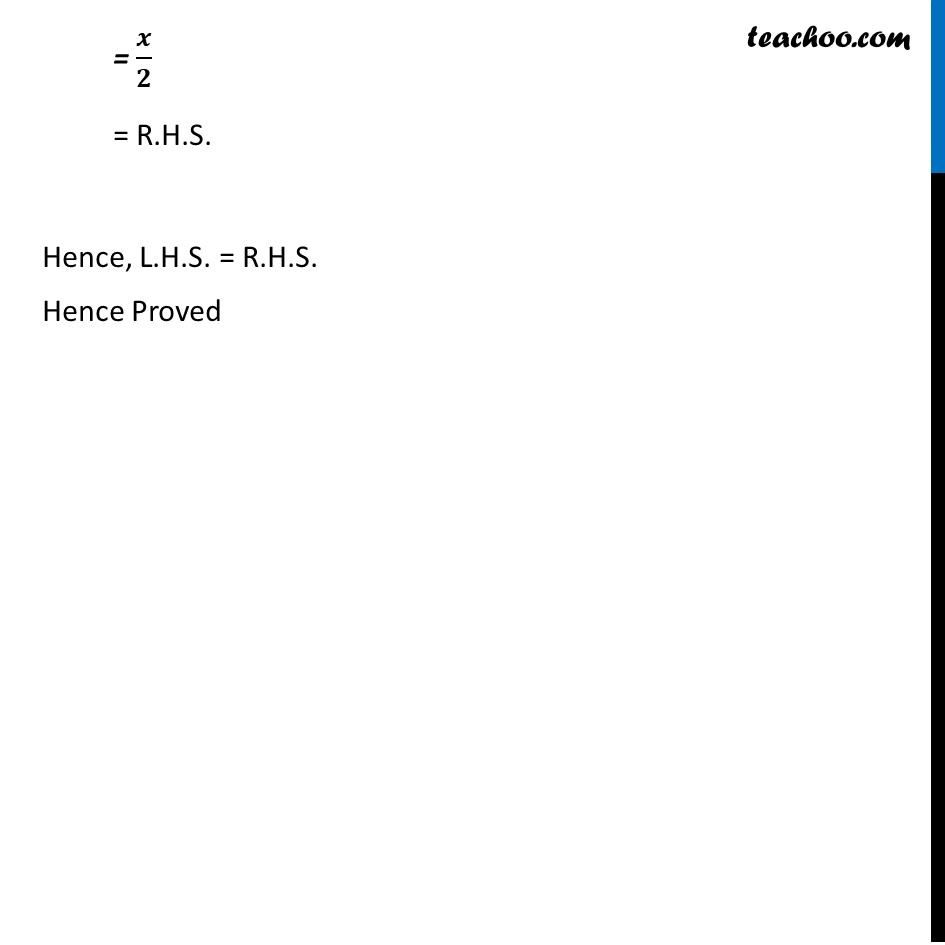1. Chapter 2 Class 12 Inverse Trigonometric Functions (Term 1)
2. Serial order wise
3. Miscellaneous

Transcript

Misc 10 Prove cot−1 ((√(1 + sin⁡〖x 〗 ) + √(1 − sin⁡x ))/(√(1 +〖 sin〗⁡x ) − √(1 − sin⁡x ))) = 𝑥/2 , x ∈ (0, 𝜋/4) First, finding √(1+sin⁡𝑥 ) & √(1−sin⁡𝑥 ) separately We know that sin 2x = 2 sin x cos x Replace x by 𝑥/2 sin (2𝑥/2) = 2 sin 𝑥/2 cos 𝑥/2 Adding 1 both sides 1 + sin x = 1 + 2 sin 𝑥/2 cos 𝑥/2 1 + sin x = sin2 𝑥/2 + cos2 𝑥/2 + 2sin 𝑥/2 cos 𝑥/2 1 + sin x = (sin 𝑥/2 + cos 𝑥/2)2 √(𝟏+𝒔𝒊𝒏⁡𝒙 ) = sin 𝒙/𝟐 + cos 𝒙/𝟐 As sin2 x + cos2 x = 1 sin2 𝑥/2 + cos2 𝑥/2 = 1 We know that sin 2x = 2sin x cos x Replace x by 𝑥/2 sin 2𝑥/2 = 2sin 𝑥/2 cos 𝑥/2 sin x = 2sin 𝑥/2 cos 𝑥/2 Multiply by –1 on both sides And then, Adding 1 both sides 1 – sin x = 1 – 2 sin 𝑥/2 cos 𝑥/2 1 - sin x = cos2 𝑥/2 + sin2 𝑥/2 – 2sin 𝑥/2 cos 𝑥/2 1 – sin x = (cos (𝑥 )/2 – sin 𝑥/2)2 √(𝟏 −𝒔𝒊𝒏⁡𝒙 ) = (cos (𝒙 )/𝟐 – sin 𝒙/𝟐) As sin2 x + cos2 x = 1 sin2 𝑥/2 + cos2 𝑥/2 = 1 Therefore, cot−1 ((√(1 + sin⁡x ) + √(1 − sin⁡x ))/(√(1 + sin⁡x ) − √(1 −〖 sin〗⁡x ))) = cot−1 ((〖𝐬𝐢𝐧 〗⁡〖𝒙/𝟐〗 + 〖𝒄𝒐𝒔 〗⁡〖𝒙/𝟐〗 + 〖𝒄𝒐𝒔 〗⁡〖𝒙/𝟐〗 − 〖𝒔𝒊𝒏 〗⁡〖𝒙/𝟐〗 )/(〖𝒔𝒊𝒏 〗⁡〖𝒙/𝟐〗 + 〖𝒄𝒐𝒔 〗⁡〖𝒙/𝟐〗 − (〖𝒄𝒐𝒔 〗⁡〖𝒙/𝟐〗 − 〖𝒔𝒊𝒏 〗⁡〖𝒙/𝟐〗 ) )) = cot−1 ((〖sin 〗⁡〖𝑥/2〗 − 〖sin 〗⁡〖𝑥/2〗 + 〖cos 〗⁡〖𝑥/2〗 + 〖cos 〗⁡〖𝑥/2〗)/(〖cos 〗⁡〖𝑥/2〗 − 〖cos 〗⁡〖𝑥/2〗 + 〖sin 〗⁡〖𝑥/2〗 + 〖sin 〗⁡〖𝑥/2〗 )) = cot−1 ((2 cos x/2 )/〖2 sin〗⁡〖 x/2 〗 ) = cot−1 ("cot " 𝐱/𝟐) = 𝒙/𝟐 = R.H.S. Hence, L.H.S. = R.H.S. Hence Proved

Miscellaneous

Chapter 2 Class 12 Inverse Trigonometric Functions (Term 1)
Serial order wise